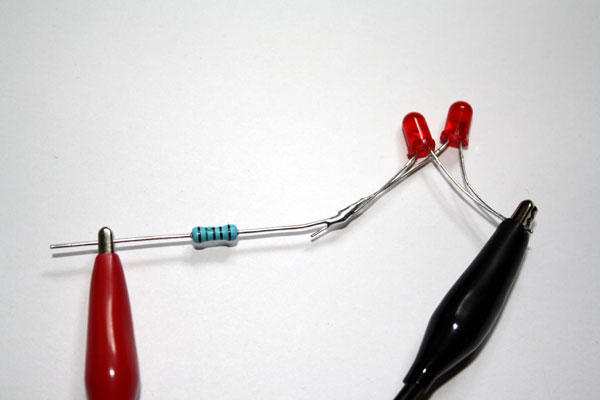# How to wire multiple LEDs

Basic electronics part 4Series wiring

In the example below, I have wired two LEDs end-to-end. This is know as wiring in seriesThe anode of the LED on the left is wired to the resistor as before – but the cathode is wired to the anode of the LED on the right. This is because from the point of view of the LED on the right it’s anode is going to the highest potential difference presented to it, and it’s cathode is going to the lowest. This is also true for the LED on the left as it cannot see the the rest of the circuit. Essentially current can only flow in one direction through the LEDs so they must both be pointing in the same direction.

These specific LEDs have a rating of 2 volts at 20mA, as before this means that if the potential difference across them is 2 volts, then they will only draw a current of 20mA. As they are wired in series the potential difference across them will be the sum of both, so the current flow will be 20mA through the LEDs when the potential difference across both of them together is 4 volts.

Our battery is again 5 volts, so this time we only need to drop one volt across the resistor, with a current flow of 20mA. Using Ohms law:

R = V/I

R = 1/0.02

R = 50

So our resistor, which is also wired in series, needs to be 50 Ohms

Parallel wiring

In the example below, I have wired both the anodes of the LEDs together, and both the cathodes of the LEDs together. This is know as wiring in parallel. There is one resistor in series with both LEDs:This time our hose pipe where the LEDs are wired is effectively twice and thick, so twice as much current can flow. However the potential difference across the LEDs must remain at 2 volts in order for the correct amount of current to flow through each.

So this time, we still need 2 volts across the LEDs, but our battery is 5 volts, so we need to drop 3 volts. However, our current is now 40mA, so using Ohms law:

R = V/I

R = 3/0.04

R = 75

So our resistor, which is wired in series with both LEDs, needs to be 75 Ohms.